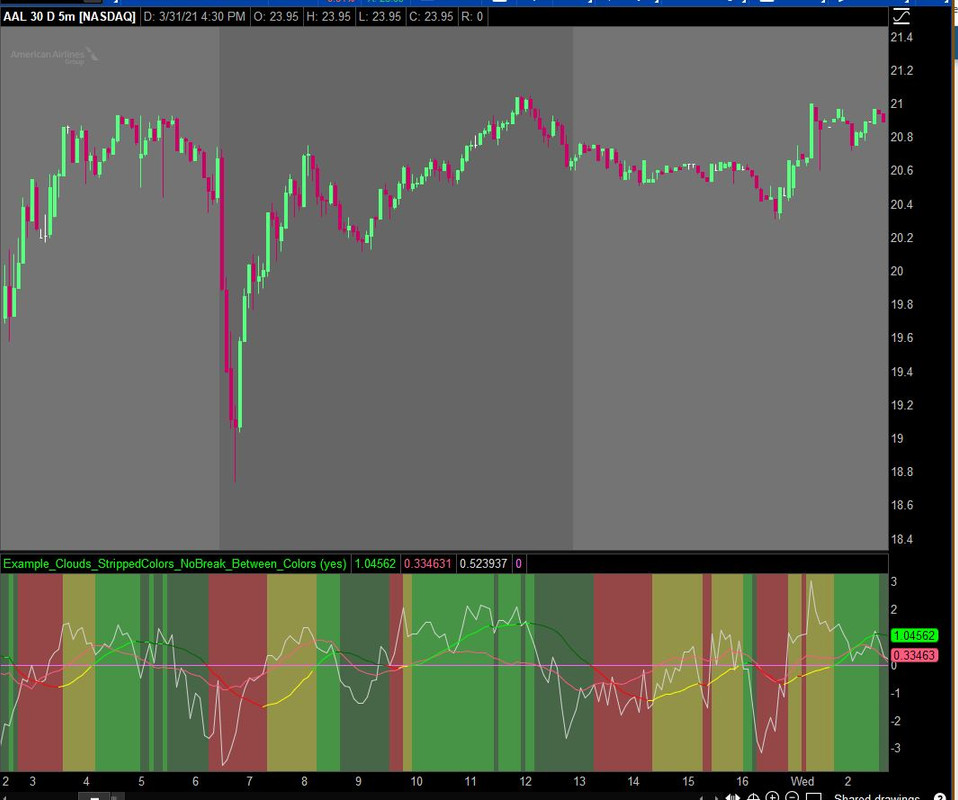# Adding Vertical Cloud background to lower study - Z Score

#### BBDPDC

##### New member
VIP
Hello folks

Feel a bit silly for asking but I can't work it out hence falling on the mercy of the group for help.

I grabbed the ZScore lower study a while ago and have updated to just show a plotted line but with positive/negative colours to indicate trend (I find this traffic light colouring suits my simple brain).

I had an idea that I would combine this study as a background on a MACD_BB lower as I use the ZScore plot as a confirmation only and want to save space/simplify the chart.....so the question is, how do I covert my line colouring to a vertical cloud background. I got as far as the below but struggling with the code that includes the infinity statements to make it vertical and getting the 4 colours in that I wanted....is that even possible?

I don't remember where i got the original code from so thanks to the author and apologies if you think I've butchered the code unnecessarily.Code:
``````#Computes and plots the Zscore
def ZavgLength = 20 ;
def oneSD = StDev(close, 20);
def avgClose = SimpleMovingAvg(close, 20);
def ofoneSD = oneSD * close;
def Zscorevalue = ((close - avgClose) / oneSD);
def avgZv = Average(Zscorevalue, 20);
def Zscore = ((close - avgClose) / oneSD);
# ########################################################
# charting & formatting:
declare lower;
#This is an optional plot that will display the momentum of the Z-Score average
DefineGlobalColor("LabelGreen",  CreateColor(0, 165, 0)) ;
plot pavgZscore = Average(Zscorevalue, ZavgLength);
plot momZAvg = (avgZv - avgZv);
plot pZscore = Zscore;
plot zero = 0;

def pos1         = pavgZscore >= 0;
def neg1         = pavgZscore < 0;
def up1        = pavgZscore >= pavgZscore;
def dn1         = pavgZscore < pavgZscore;

def PosUp1 = pos1 and up1;
def PosDn1 = pos1 and dn1;
def NegDn1 = neg1 and dn1;
def NegUp1 = neg1 and up1;

pavgZscore.AssignValueColor(if PosUp1 then Color.GREEN else if PosDn1 then Color.DARK_GREEN else if NegDn1 then Color.RED else if NegUp1 then Color.YELLOW else Color.YELLOW);
zero.SetDefaultColor(GetColor(0));

AddCloud(posup1 or negup1, negdn1 or posdn1, Color.light_GREEN, Color.light_RED);

#=====================================``````

#### SleepyZ

##### Active member
Hello folks

Feel a bit silly for asking but I can't work it out hence falling on the mercy of the group for help.

I grabbed the ZScore lower study a while ago and have updated to just show a plotted line but with positive/negative colours to indicate trend (I find this traffic light colouring suits my simple brain).

I had an idea that I would combine this study as a background on a MACD_BB lower as I use the ZScore plot as a confirmation only and want to save space/simplify the chart.....so the question is, how do I covert my line colouring to a vertical cloud background. I got as far as the below but struggling with the code that includes the infinity statements to make it vertical and getting the 4 colours in that I wanted....is that even possible?

I don't remember where i got the original code from so thanks to the author and apologies if you think I've butchered the code unnecessarily.

See if this helps.
Code:
``````#Computes and plots the Zscore
def ZavgLength = 20 ;
def oneSD = StDev(close, 20);
def avgClose = SimpleMovingAvg(close, 20);
def ofoneSD = oneSD * close;
def Zscorevalue = ((close - avgClose) / oneSD);
def avgZv = Average(Zscorevalue, 20);
def Zscore = ((close - avgClose) / oneSD);
# ########################################################
# charting & formatting:
declare lower;
#This is an optional plot that will display the momentum of the Z-Score average
DefineGlobalColor("LabelGreen",  CreateColor(0, 165, 0)) ;
plot pavgZscore = Average(Zscorevalue, ZavgLength);
plot momZAvg = (avgZv - avgZv);
plot pZscore = Zscore;
plot zero = 0;

def pos1         = pavgZscore >= 0;
def neg1         = pavgZscore < 0;
def up1        = pavgZscore >= pavgZscore;
def dn1         = pavgZscore < pavgZscore;

def PosUp1 = pos1 and up1;
def PosDn1 = pos1 and dn1;
def NegDn1 = neg1 and dn1;
def NegUp1 = neg1 and up1;

pavgZscore.AssignValueColor(if PosUp1 then Color.GREEN else if PosDn1 then Color.DARK_GREEN else if NegDn1 then Color.RED else if NegUp1 then Color.YELLOW else Color.YELLOW);
zero.SetDefaultColor(GetColor(0));

#AddCloud(posup1 or negup1, negdn1 or posdn1, Color.light_GREEN, Color.light_RED);

def cond1 = if PosUp1
then Double.POSITIVE_INFINITY
else Double.NEGATIVE_INFINITY;
def cond2 = if Posdn1
then Double.POSITIVE_INFINITY
else Double.NEGATIVE_INFINITY;
def cond3 = if NegDn1
then Double.POSITIVE_INFINITY
else Double.NEGATIVE_INFINITY;
def cond4 = if NegUp1
then Double.POSITIVE_INFINITY
else Double.NEGATIVE_INFINITY;
input showclouds = yes;
AddCloud(if showclouds
then cond1
else double.nan,
cond2,
Color.GREEN, Color.Dark_Green);
AddCloud(if showclouds
then cond3
else double.nan,
cond4,
Color.Red, Color.Yellow);

#=====================================``````•Chuck, purePlay and BBDPDC

#### BBDPDC

##### New member
VIP
@SleepyZ Thank you! This is exactly what I was trying to do!